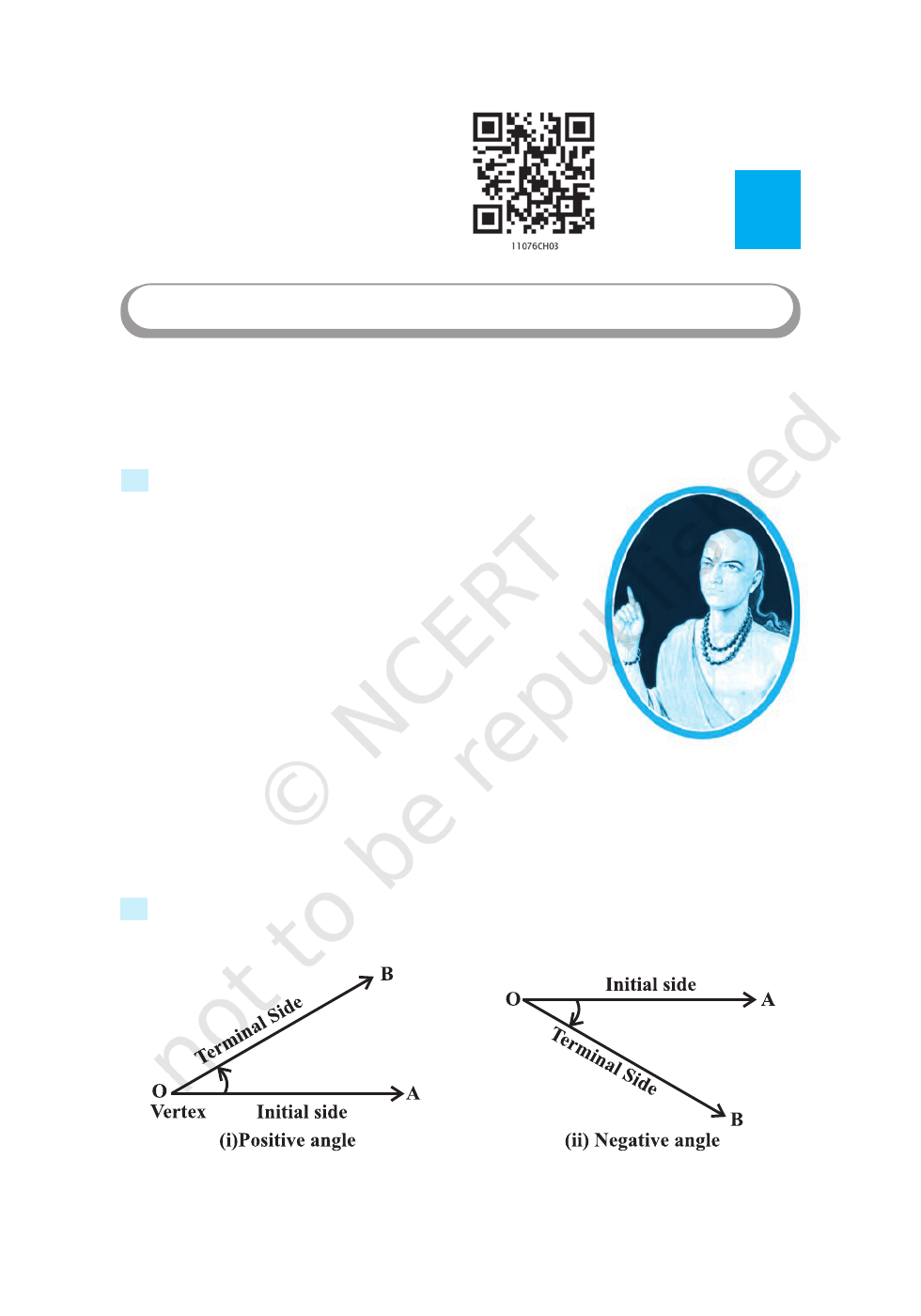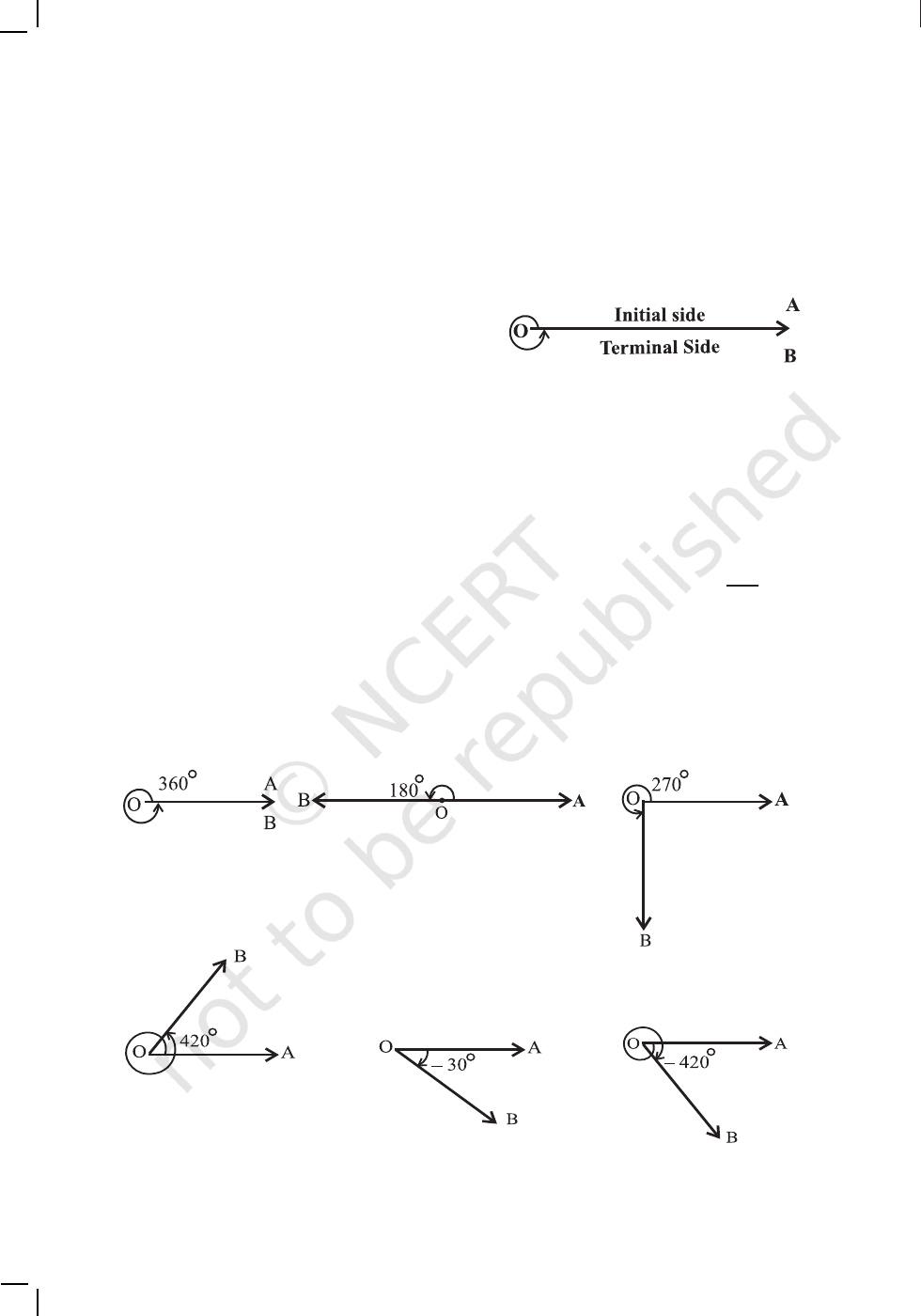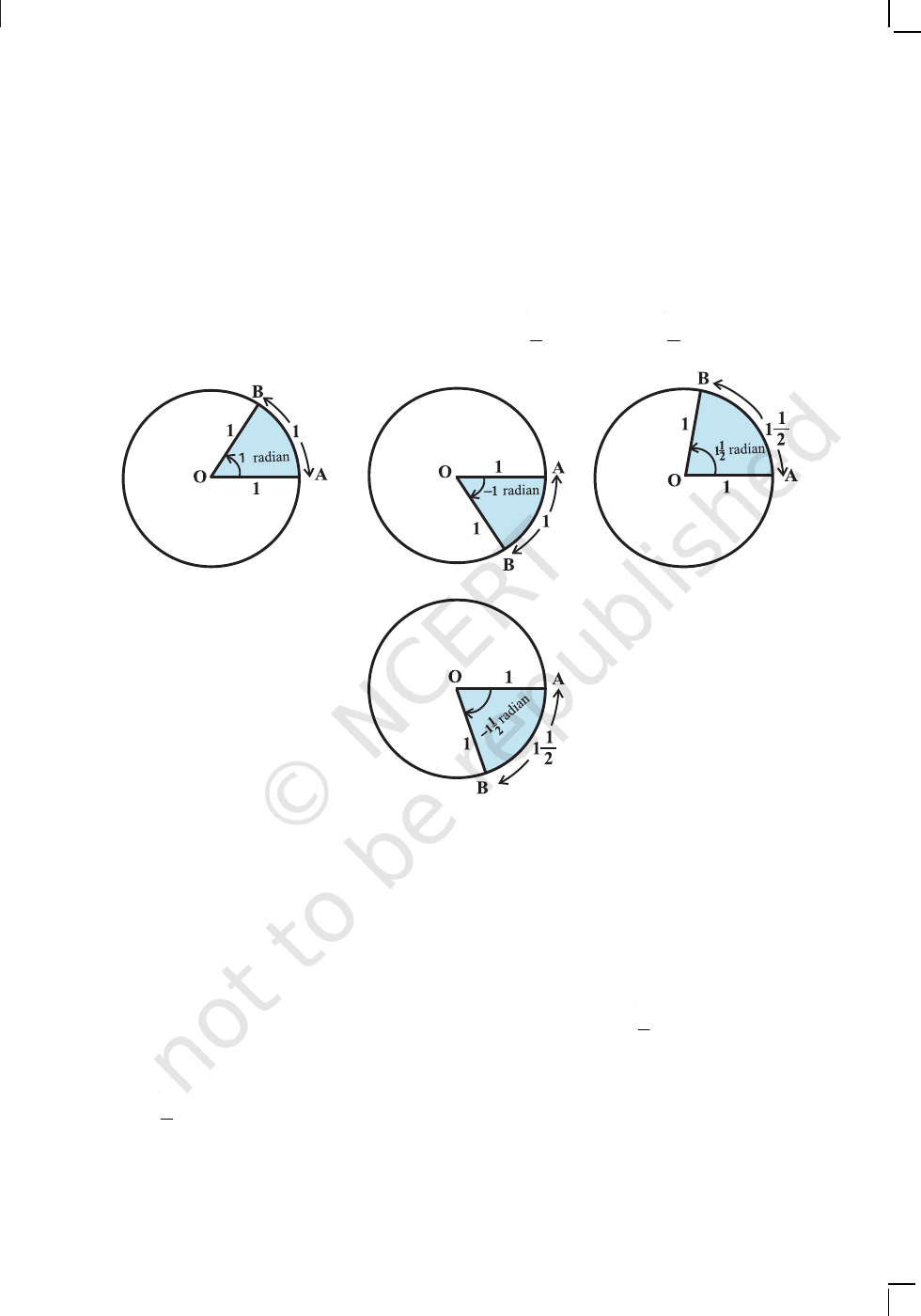vA mathematician knows how to solve a problem,
he can not solve it. – MILNE v
3.1 Introduction
The word ‘trigonometry’ is derived from the Greek words
trigon and ‘metron and it means ‘measuring the sides of
a triangle’. The subject was originally developed to solve
geometric problems involving triangles. It was studied by
sea captains for navigation, surveyor to map out the new
lands, by engineers and others. Currently, trigonometry is
used in many areas such as the science of seismology,
designing electric circuits, describing the state of an atom,
predicting the heights of tides in the ocean, analysing a
musical tone and in many other areas.
In earlier classes, we have studied the trigonometric
ratios of acute angles as the ratio of the sides of a right
angled triangle. We have also studied the trigonometric identities and application of
trigonometric ratios in solving the problems related to heights and distances. In this
Chapter, we will generalise the concept of trigonometric ratios to trigonometric functions
and study their properties.
3.2 Angles
Angle is a measure of rotation of a given ray about its initial point. The original ray is
Chapter
3
TRIGONOMETRIC FUNCTIONS
Arya Bhatt
(476-550)
Fig 3.1
Vertex
2020-2150 MATHEMATICS
called the initial side and the final position of the ray after rotation is called the
terminal side of the angle. The point of rotation is called the vertex. If the direction of
rotation is anticlockwise, the angle is said to be positive and if the direction of rotation
is clockwise, then the angle is negative (Fig 3.1).
The measure of an angle is the amount of
rotation performed to get the terminal side from
the initial side. There are several units for
measuring angles. The definition of an angle
suggests a unit, viz. one complete revolution from the position of the initial side as
indicated in Fig 3.2.
This is often convenient for large angles. For example, we can say that a rapidly
spinning wheel is making an angle of say 15 revolution per second. We shall describe
two other units of measurement of an angle which are most commonly used, viz.
3.2.1
Degree measure
If a rotation from the initial side to terminal side is
th
1
360
of
a revolution, the angle is said to have a measure of one degree, written as 1°. A degree is
divided into 60 minutes, and a minute is divided into 60 seconds . One sixtieth of a degree is
called a minute, written as 1, and one sixtieth of a minute is called a second, written as 1.
Thus, 1° = 60′, 1 = 60
Some of the angles whose measures are 360°,180°, 270°, 420°, – 30°, – 420° are
shown in Fig 3.3.
Fig 3.2
Fig 3.3
2020-21TRIGONOMETRIC FUNCTIONS 51
There is another unit for measurement of an angle, called
the radian measure. Angle subtended at the centre by an arc of length 1 unit in a
unit circle (circle of radius 1 unit) is said to have a measure of 1 radian. In the Fig
3.4(i) to (iv), OA is the initial side and OB is the terminal side. The figures show the
1
2
1
2
(i)
(ii)
(iii)
Fig 3.4 (i) to (iv)
(iv)
We know that the circumference of a circle of radius 1 unit is 2π. Thus, one
complete revolution of the initial side subtends an angle of 2π radian.
More generally, in a circle of radius r, an arc of length r will subtend an angle of
1 radian. It is well-known that equal arcs of a circle subtend equal angle at the centre.
Since in a circle of radius r, an arc of length r subtends an angle whose measure is 1
radian, an arc of length l will subtend an angle whose measure is
l
r